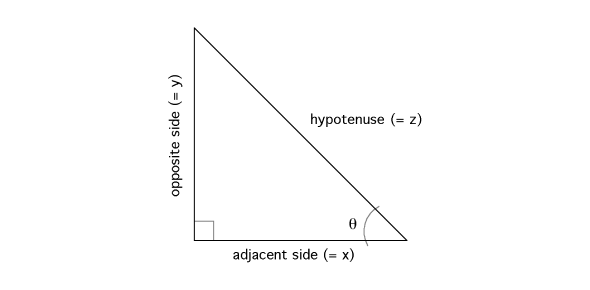# Quiz: Can You Pass The Triangle Trigonometry Test?

7 QuestionsSettingsCan You Pass The Triangle Trigonometry Test? There are a lot of people who have difficulty in solving trigonometry questions, and the quiz below is perfectly prepared for them. If you are in this group of students, be sure to give it a shot and be a step closer to perfecting your ability to solving said math problems.

Related Topics
• 1.
Find the sides of this triangle.
• A.

M = 37.6, N = 13.7

• B.

M = 13.7, N = 37.6

• C.

M = 117, N = 42.6

• D.

M = 42.6, N = 117

• 2.
Find the missing values on this triangle.
• A.

K = 75, Y = 41.4

• B.

K = 39.7, Y = 48.6

• C.

K = 39.7, Y = 41.4

• D.

K = 75, Y = 48.6

• 3.
Which trig function equals the sin ?
• A.

B / c

• B.

A / c

• C.

A / b

• D.

B / a

• 4.
Which trig function equals the tan ?
• A.

B / c

• B.

A / c

• C.

A / b

• D.

C / b

• 5.
Which trig function equals the cos ?
• A.

B / c

• B.

C / a

• C.

A / c

• D.

B / a

• 6.
Find the missing values on this triangle.
• A.

T = 23.0, Y = 29.7

• B.

T = 23.0, Y = 34.8

• C.

T = 32.2, Y = 29.7

• D.

T = 32.2, Y = 34.8

• 7.
Find the missing values on this triangle.
• A.

V = 31.1, W = 112

• B.

V = 29.0, W = 7.7

• C.

V = 31.1, W = 8.0

• D.

V = 116, W = 112